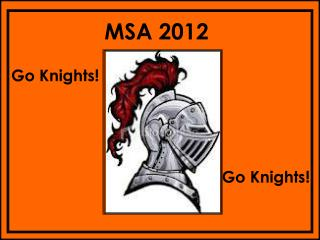DownloadDownload PresentationMSA 2012

MSA 2012

Télécharger la présentationMSA 2012

- - - - - - - - - - - - - - - - - - - - - - - - - - - E N D - - - - - - - - - - - - - - - - - - - - - - - - - - -
Presentation Transcript

1. MSA 2012 Go Knights! Go Knights!

2. 1 AC is a ____________. F A E B D C

4. 2 This figure shows a _________.

6. 3 This figure shows a _____________.

8. 4 This figure shows an _________.

10. 5 Angle ABC equals 80 degrees A D B C If you bisect angle ABC, then angle ABD equals ______.

11. Answer: 40 degrees 80 divided by 2 = 40 degrees

12. 6 This figure shows the ________ of a circle.

14. 7 This figure shows the _________ of a circle.

16. 8 If the diameter of a circle is 14 cm then the radius is _____ cm.

17. Answer: 7 cm 14 divided by 2 = 7 cm

18. 9 If the radius of a circle is 3.4 cm then the diameter is _____ cm.

19. Answer: 6.8 cm 3.4 x 2 = 6.8 cm

20. 10 This graph is __________.

22. 11 This graph is _________.

24. 12 This graph has _____________.

26. 13 This line shows a graph that is _______________.

28. 14 This line shows a graph that is _______________.

30. 15 This line shows a graph that has _______________.

32. 16 Name this shape.

34. 17 Name this shape.

36. 18 Find the area of this square. 7 cm

37. Answer: 49 square cm 7 cm x 7 cm = 49 square cm

38. 19 Find the area of this rectangle. 5 in. 8 in.

39. Answer: 40 square in. 8 in. x 5 in. = 40 square in.

40. 20 Find the area of this triangle. 8 ft. 10 ft.

41. Answer: 40 square ft. 10 x 8 = 80 80 divided by 2 = 40 square ft.

42. 21 Find the area of this triangle. 12 ft. 5 ft. 9 ft 4 ft

43. Answer: 10 square ft. 5 ft x 4 ft. = 20 square ft. 20 divided by 2 = 10 square ft.

44. 22 If the area of this square is 64 square cm, what is the length of one side?

45. Answer: 8 cm Because 8 cm x 8 cm = 64 square cm

46. 23 If the perimeter of this square is 28 inches, what is the length of one side?

47. Answer: 7 cm Because 28 divided by 4 = 7 cm or Because 7 + 7 + 7 + 7 = 28 cm

48. 24 If the area of this rectangle is 42 square inches, what is the width? ? 7 in.

49. Answer: 6 in. 42 divided by 7 = 6 in. or 6 in. x 7 in. = 42 square in.

50. 25 If the perimeter of this rectangle is 20 inches, what is the length? 3 in. ?